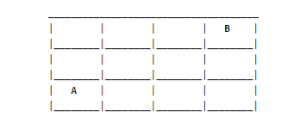# Find the different paths possible

1,265.3K Views

Consider a rectangular grid of 4×3 with lower  left corner named as A and upper right corner named B. Suppose that starting point is A and you can move one step up(U) or one step right(R) only. This is continued until B is reached. How many different paths from A to B possible ?Now let’s look at some sample paths we can figure out by inspection.

If we start at A and move towards B, we find we can follow the path

RRRUU

(where R = Right one unit, U = Up one unit),

UURRR,
RURUR,
RRUUR,

and so on.

By analyzing our good routes, we see that every good route consists of 5 moves and we have 3 R moves and 2 U moves. We canuse this to generalize a formula to find the number of possible routes.

Since as we’ve shown, order does not matter in our paths (we can have an R in any place of our 5 moves), we can use our combination formula:

C(N,R) = N!/(N-R)! * R!

The number of how many good routes we have can be found by finding how many combinations of 3 R’s we can have in our 5 moves, so we want to calculate:

C(5,3) = 5!/(5-3)! * 2! = 10